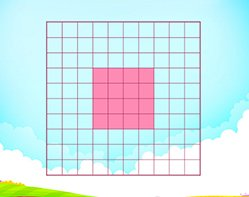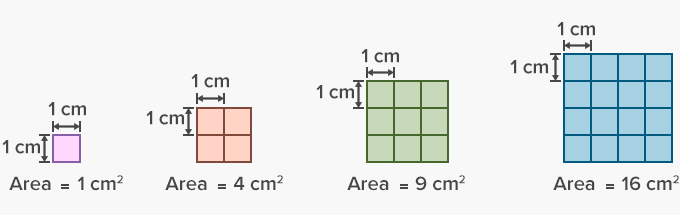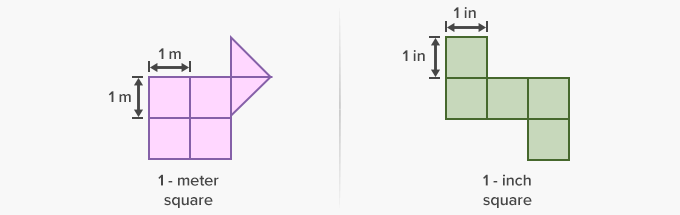# Area - Definition with Examples

The Complete K-5 Math Learning Program Built for Your Child

• 30 Million Kids

Loved by kids and parent worldwide

• 50,000 Schools

Trusted by teachers across schools

• Comprehensive Curriculum

Aligned to Common Core

## Area GamesArea

Use unit squares to understand the concept of area and find area for different two dimensional shapes.

Covers Common Core Curriculum 3.MD.7

## What is Area?

In geometry, the area can be defined as the space occupied by a flat shape or the surface of an object.
The area of a figure is the number of unit squares that cover the surface of a closed figure. Area is measured in square units such as square centimteres, square feet, square inches, etc.

The area of the squares below, with unit squares of sides 1 centimeter each, will be measured in square centimeters (cm²).Here, the area of the shapes below will be measured in square meters (m²) and square inches (in²).The origin of the word area is from ‘area’ in Latin, meaning a vacant piece of level ground. The origin further led to an irregular derivation of area as ‘a particular amount of space contained within a set of boundaries’.

We often find the area of the room floor to determine the size of the carpet to be bought. Covering the floor with tiles, covering the wall with paint or wallpaper or building a swimming pool are other examples, where in the area is computed.

Common simple shapes and polygons have their own formulae for calculating area. Here’s how area of common 2-D or Two-dimensional shapes is computed:
 Two-Dimensional Geometric Shapes:
 Name of the shape: Picture of shape: Area Formula: CircleArea = πr², where r is the radius. TriangleArea =bh, where b is base, And h is height. SquareArea =l × l, where l is the length of each side. RectangleArea = l × w, where l is length and w is width. ParallelogramArea = b × h,  where b is base, and h is perpendicular height. TrapeziumArea=(a +b)h , where a and b are lengths of the parallel sides, and h is perpendicular height.

In real life, not every plane figure can be clearly classified as a rectangle, square or a triangle. To find the area of a composite figure which consists of more than one shape, we need to find the sum of the area of both or all the shapes forming the composite figure.

The area of the outside surface of a solid or three-dimensional shape is called Surface Area. For instance, a rectangular prism has 6 rectangular bases and lateral faces. So, the total surface area is the sum of the areas of all the 6 rectangles.

 Fun Facts Among all the shapes with same perimeter, a circle has the largest area.

###Let's sing!

To plant vegetables in the garden backside,
Find the area of the field – the whole of inside.
Multiply its length with how much it is wide,
And, that’s the area formula, you have applied!

###Let's do it!

Instead of handing out math worksheets on the area to your children, involve them in home improvement projects. Inform them about the room that you are considering to paint. Ask them to calculate the total surface area of the walls, in order to find how much paint is required.

Discuss how all walls of the room may be different and thus, taking overall measurements and then subtracting the area of the door, windows or book shelves, will help in estimating the amount of paint needed.
You can also ask to consider finding the total surface area of a present that they wrapping in order to find the amount of wrapping paper required.

###Related math vocabulary

Won Numerous Awards & Honors